• ## dfs和bfs遍历生成树

千次阅读 2019-12-13 11:34:18
#include<iostream>...void dfs(char a[],int arc[],int s[],int n,int x){ for(int i=0;i<n;i++){ if(arc[x][i]&&s[x]==0){ cout<<a[x]; s[x]=1; dfs(a...
dfs

void dfs(char s[],int arc[],int v[],int n,int x){
//顶点字符串、边、已经遍历过的顶点、顶点个数、当前遍历的顶点的下标
v[x]=1;
for(int i=0;i<n;i++){
if(arc[x][i]&&!v[i]){//顶点i与顶点x有边且i尚未遍历
dfs(s,arc,v,n,i);//遍历顶点x的每一个邻接点
}
}
}

bfs

void bfs(char s[],int arc[],int v[],int n,int x){//对顶点x广度优先遍历
int front=-1,rear=-1;
int q;//存下标！！
q[++rear]=x;//a[x]入队
v[x]=1;
while(front!=rear){
front++;
for(int i=0;i<n;i++){//存入front的邻接点(出队的那个顶点的邻接点)
if(!v[i]&&arc[q[front]][i]){//不是front，是q[front]:下标！！
//顶点i尚未入队且i与顶点q[front]有边
q[++rear]=i;//i入队
v[i]=1;//标记i已入队
}
}
}

}

实例
广度优先生成树
Time Limit: 1000/2000 MS (C/Others)   Memory Limit: 32768/32768 K (C/Others)
Problem Description:
设有一连通无向图，其顶点值为字符型并假设各值互不相等，采用邻接矩阵表示法存储表示。利用BFS算法求其广度优先生成树（从下标0的顶点开始遍历），并在遍历过程中输出广度优先生成树的每一条边。
Input:
有多组测试数据，每组数据的第一行为两个整数n和e，表示n个顶点和e条边（0<n<20）；第二行为其n个顶点的值，按输入顺序进行存储；后面有e行，表示e条边的信息，每条边信息占一行，包括边所依附的顶点下标i和j，数据之间用空格隔开。
Output:
输出广度优先生成树的每一条边，每条边信息之后均有一空格，每组输出占一行，具体格式见样例。
Sample Input:
4 4
ABCD
0 1
0 3
1 2
1 3
Sample Output:
(A,B) (A,D) (B,C)
Hint:
Source:
代码
#include<iostream>
using namespace std;
void dfs(char a[],int arc[],int s[],int n,int x){
cout<<a[x];
s[x]=1;
for(int i=0;i<n;i++){
if(arc[x][i]&&s[i]==0){
arc[x][i]=arc[i][x]=0;
dfs(a,arc,s,n,i);
}
}
}
void bfs(char a[],int arc[],int s[],int n,int x){
int front=-1,rear=-1;
int q;//存下标
q[++rear]=x;//a[x]入队
s[x]=1;
while(front!=rear){
cout<<a[q[++front]];
for(int i=0;i<n;i++){//存入front的邻接点(出队的那个的邻接点)
//	cout<<"arc["<<q[front]<<"]["<<i<<"]:"<<arc[front][i]<<endl;
if(!s[i]&&arc[q[front]][i]){
//		cout<<"i:"<<i<<endl;
q[++rear]=i;
s[i]=1;
}
}
}

}
int main(){
int n,e;
while(cin>>n>>e){
char s;
int arc={0};
cin>>s;
int a,b;
while(e--){
cin>>a>>b;
arc[a][b]=arc[b][a]=1;
}
int ss={0};
dfs(s,arc,ss,n,0);
cout<<endl;
}
return 0;
}




展开全文数据结构
• 图分为：无向图有向图 图的遍历：深度优先遍历（Depth-First Search，DFS广度优先遍历（Breadth-First Search，BFS）。 深度优先遍历广度优先遍历参考博客：https://segmentfault.com/a/1190000002685939
UDG：无向图

图分为：无向图（UDG）和有向图（DG）
图的表示方法：邻接表和邻接矩阵。邻接表表示参考：http://blog.csdn.net/linxinyuluo/article/details/6847851
图的遍历：深度优先遍历（Depth-First Search，DFS）和广度优先遍历（Breadth-First Search，BFS）。
深度优先遍历类似与树的先序遍历，广度优先遍历类似与树的层次遍历
深度优先遍历和广度优先遍历参考博客：https://segmentfault.com/a/1190000002685939
深度优先遍历可以通过递归或者非递归，其中非递归要采用栈的形式存储结点；广度优先遍历一般通过非递归，需要通过队列的形式存储结点。

c++实现：
（1）邻接矩阵无向图的DFS和BFS：https://github.com/wangkuiwu/datastructs_and_algorithm/blob/master/source/graph/iterator/udg/cplus/MatrixUDG.cpp
（2）邻接表无向图的DFS和BFS：https://github.com/wangkuiwu/datastructs_and_algorithm/blob/master/source/graph/iterator/udg/cplus/ListUDG.cpp
（3）邻接矩阵有向图的DFS和BFS：https://github.com/wangkuiwu/datastructs_and_algorithm/blob/master/source/graph/iterator/dg/cplus/MatrixDG.cpp
（4）邻接表有向图的DFS和BFS：https://github.com/wangkuiwu/datastructs_and_algorithm/blob/master/source/graph/iterator/dg/cplus/ListDG.cpp

图的生成树：
G的生成树是G的极小连通子图，图的生成树不唯一，从不同的顶点出发进行遍历，可以得到不同的生成树。
1、DFS生成树：
2、BFS生成树：BFS生成树在G的所有生成树中的高度是最低的。
参考：http://blog.csdn.net/coslay/article/details/38716183

展开全文• DFS和BFS 1：图的DFS=的先根遍历=二叉树的先序遍历。 从无向图任意结点出发进行一次DFS即可访问所有结点，则该图是：连通图 判断一个有向图是否有环亦可用DFS 2：基于邻接矩阵的DFS时间复杂度：O(n^2),基于邻接表...
DFS和BFS
1：图的DFS=树的先根遍历=二叉树的先序遍历。
从无向图任意结点出发进行一次DFS即可访问所有结点，则该图是：连通图
判断一个有向图是否有环亦可用DFS
2：基于邻接矩阵的DFS时间复杂度：O(n^2),基于邻接表的DFS时间复杂度：O(n+e)
3：图的BFS=树的层次遍历，能够遍历所有与该结点连通的顶点，可求无向图的所有连通分量
4：基于邻接矩阵的BFS是唯一的,基于邻接表的BFS不唯一
连通性
连通度：在连通图上至少删除k个顶点才能破坏图的连通性，称k为该图的连通度
最小生成树
定义：不允许有环，从n个顶点的连通图选取n-1条权值最小的边
两种算法：Prim算法和Kruskal算法
任何一个连通图的最小生成树有一棵或者多棵，因为可能有多条权值相同的边
1：Prim：从某个顶点出发，选取与其连接的最小路径,再从被选的那个结点出发，重复以上操作，直到遍历完所有结点。
此时的图存储结构是邻接矩阵
Prin算法时间复杂度：O(n^2)， 适用于稠密网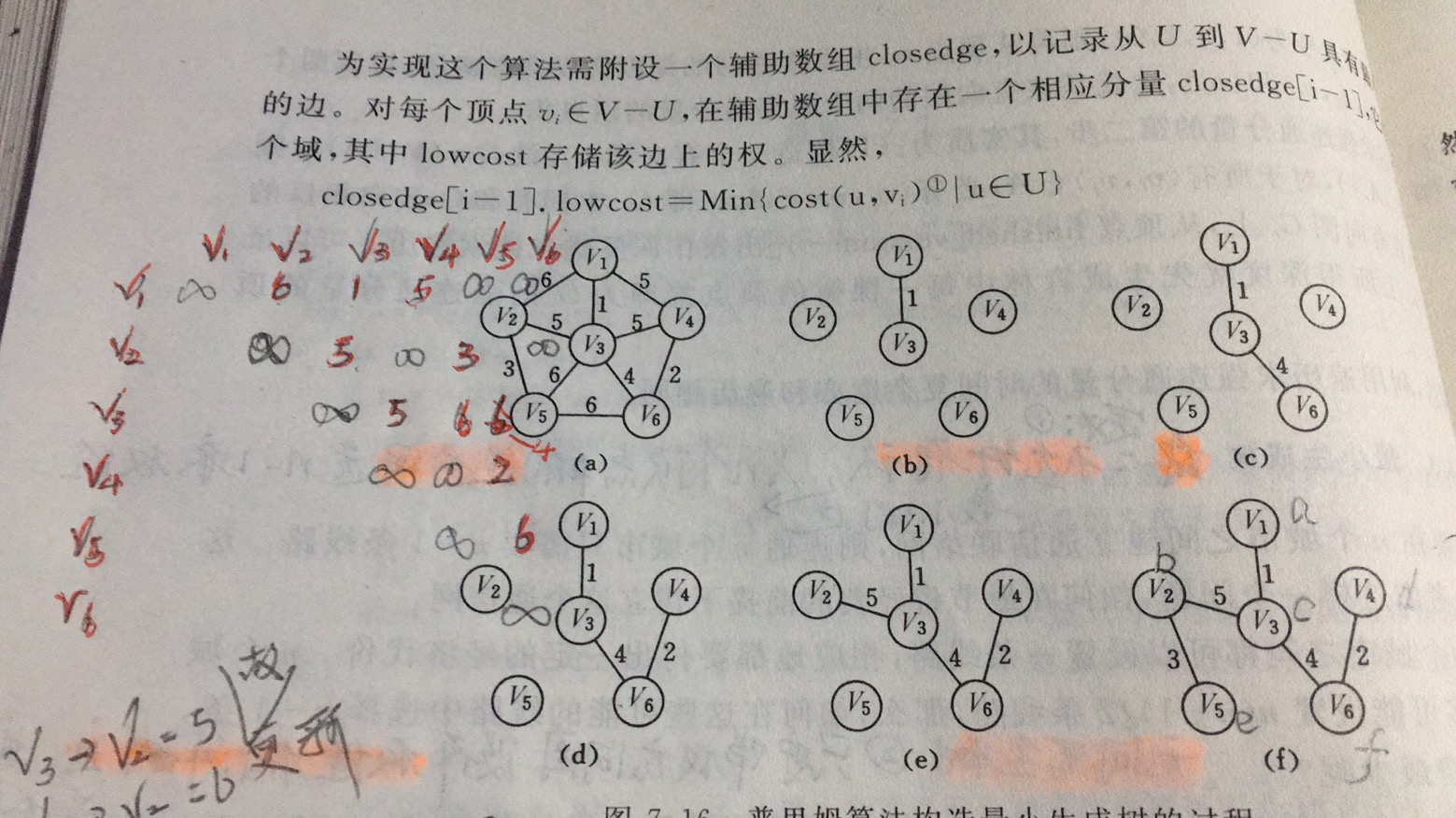#define Max_Vex 6 //最大范围
#define LostType int
typedef char  VertexType;//顶点名字的类型
typedef enum {
DG,DN,UDG,UDN//有向图，有向网，无向图 无向网
}GraphKing;

typedef struct ArcCell{
VRType  adj;//弧或者边的权值等
InfoType *Info;//该弧相关信息的指针
}ArcCell,AdjMatrix[MAX_VERTEX_MUN][MAX_VERTEX_MUN];//该矩阵的每一个元素拥有其成员adj 和 *info

typedef struct MGraph{
VertexType vexs[MAX_VERTEX_MUN];//  比如说V1 V2 V3。。。。的类型
AdjMatrix arcs;//邻接矩阵
int vexnum,arcnum;//当前顶点数和弧数
GraphKing kind;
}MGraph,*MGraphLink;

typedef struct {
int adjvex;//顶点名
LostType Lowlost;
}Prim,Closedge[Max_Vex];

int Minnum(Closedge closedge){//进来   返回下一个结点；第K个结点 最小的那个
int i,j=0,min=100;
for(i=1;i<Max_Vex;i++){
if((closedge[i].Lowlost>0)&&(min>closedge[i].Lowlost)) {
min=closedge[i].Lowlost;
printf("选好的顶点%d权值%d",i,min);
j=i;//当前矩阵最小值的下标
}
}
return j;
}

void MiniSpanTree_PRIM(MGraph G){//最小生成树的普利姆算法
//暂时约定从第一个顶点进入（开始）

int i,k=0,j=0;Closedge  closedge;
printf("入口的顶点%d",G.vexs);
for(i=1;i<G.vexnum;i++){
closedge[i].adjvex=G.vexs[i];//顶点名  坐标
closedge[i].Lowlost =G.arcs[k][i].adj;//第一个顶点与其余各顶点(不包括第一个顶点,index从0开始)的权值
} //对于无向图来说，只用遍历一行(列)就能得到weigth
closedge.Lowlost=0;//入口入U集、、下次就不比较了

for(i=1;i<G.vexnum;++i) {
k=Minnum(closedge);//最小结点的序号
printf("---->%c",G.vexs[k]);  //被选的顶点
closedge[k].Lowlost=0;//第k个顶点入U集合 下次就不用比较了
for(j=0;j<G.vexnum;++j){//更新closedge数组
if(G.arcs[k][j].adj<closedge[j].Lowlost){//重新更新closedge
closedge[j].adjvex=G.vexs[j];
closedge[j].Lowlost=G.arcs[k][j].adj;//权值
}
}
}

return 0;
}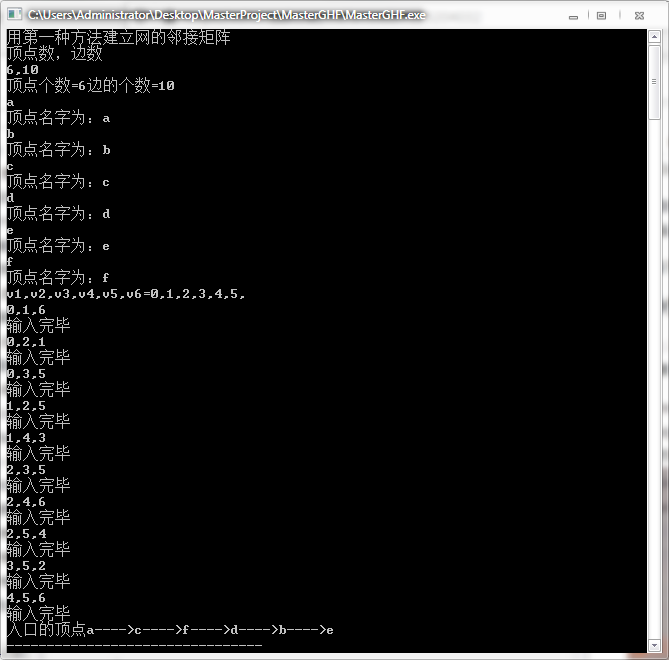2：Kruskal算法：第一步：多次选“两两权值最小且不重复的顶点相连”，如果总的顶点树目为奇数则余留一个等下次再选，第二步：重复第一步骤，直到变成连通图。时间复杂度：O(elog2e)，通常选并查集做辅助，选权值最小的原则：不能构成回路，适用于稀疏图


展开全文• 广度优先遍历(BFS) 图的广度优先遍历可定义如下：首先访问出发点v，并将其标记为已访问过；然后从v出发依次访问v的每个未被访问的邻接点w。当v的所有邻接点全部访问完后，再对v的每个邻接点w继续进行广度优先遍历，...
广度优先遍历(BFS)
图的广度优先遍历可定义如下：首先访问出发点v，并将其标记为已访问过；然后从v出发依次访问v的每个未被访问的邻接点w。当v的所有邻接点全部访问完后，再对v的每个邻接点w继续进行广度优先遍历，直至图中所有和源点v有路径相通的顶点均已被访问为止。若此时图中仍有未访问的顶点，则另选一个尚未访问的顶点为新的源点重复上述过程，直至图中所有的顶点均已被访问为止。
如下代码只考虑无向连通图，即图中任意两个顶点均有路径相连。同时由于图的广度优先遍历不唯一，以下代码对于多邻点的顶点的遍历，按顶点值由低到高进行广度优先遍历。
代码运行后，第一行输入图的顶点总数目n，顶点依次编号为1，2…n，顶点编号即对应顶点值；第二行输入图的总边数m，接下来m行输入每条边的两个顶点，以逗号分隔；最后输入遍历的始发顶点编号x。比如：下图输入顶点数8（对应的顶点为1~8），边数10和边集，始发顶点为8，得到的广度优先遍历的结果为：8 1 2 5 3 6 4 7。 程序输出广度遍历结果（空格隔开）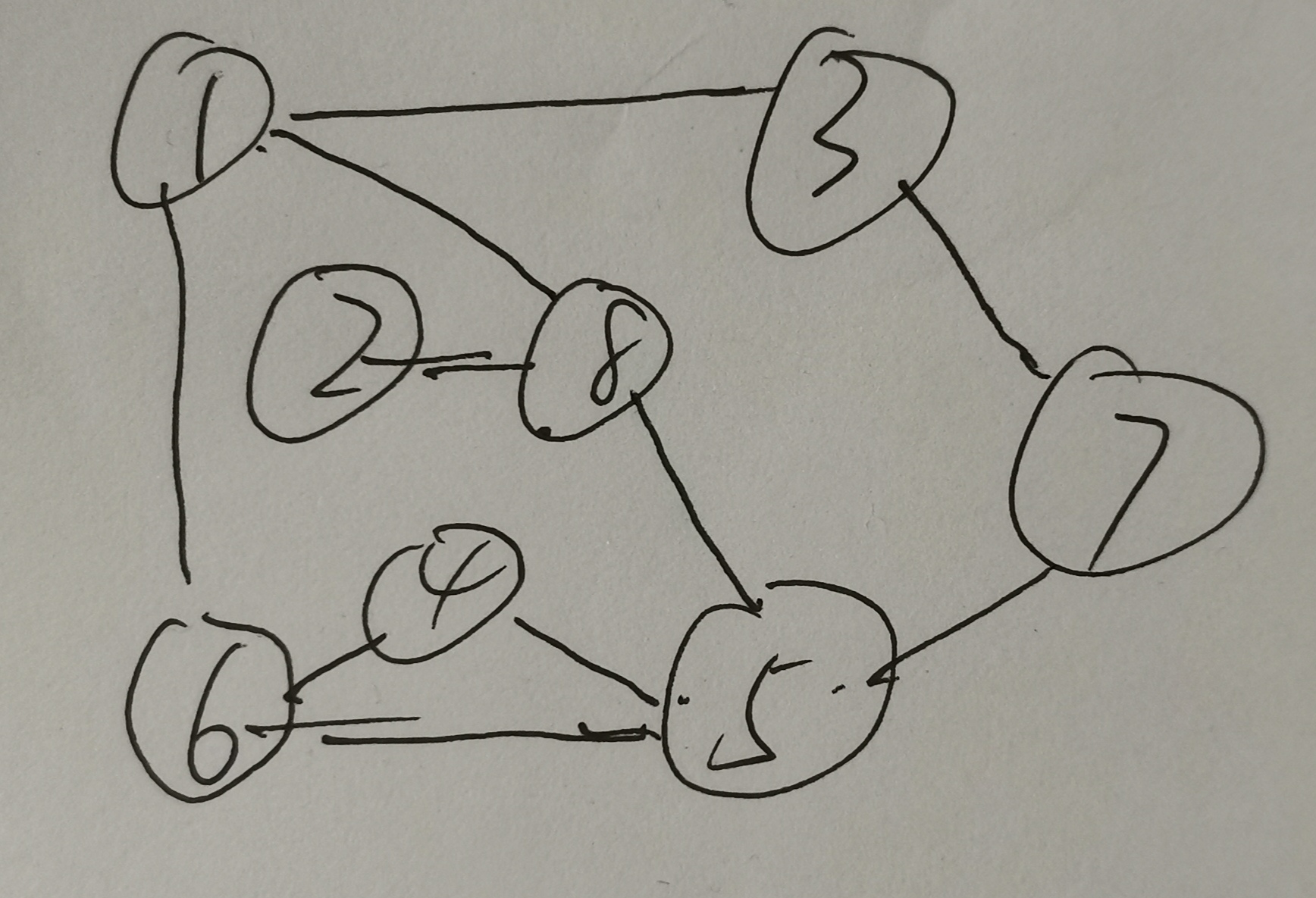class Graph(object):

def __init__(self,*args,**kwargs):
self.node_neighbors = {} # 定义顶点与邻接顶点集为字典结构
self.visited = {} # 定义已访问顶点集为字典结构

def add_nodes(self,nodelist):

for node in nodelist:
self.add_node(node)

def add_node(self,node):
if not node in self.nodes():
self.node_neighbors[node] = []

def add_edge(self,edge):
u,v = edge
if(v not in self.node_neighbors[u]) and ( u not in self.node_neighbors[v]): # 此处可添加自己指向自己的边
self.node_neighbors[u].append(v)

if(u!=v):
self.node_neighbors[v].append(u)

def nodes(self):
return self.node_neighbors.keys()

def breadth_first_search(self,root=None): # 广度优先需用到队列结构
queue = []
order = []
def bfs():
while len(queue)> 0:
node  = queue.pop(0)
self.visited[node] = True
self.node_neighbors[node].sort()
for n in self.node_neighbors[node]:
if (not n in self.visited) and (not n in queue):
queue.append(n)
order.append(n)

if root:
queue.append(root)
order.append(root)
bfs()

for node in self.nodes():
if not node in self.visited:
queue.append(node)
order.append(node)
bfs()
# print(order)
return order

if __name__ == '__main__':
g = Graph()
node_num=int(input())#顶点的数目
g.add_nodes([i+1 for i in range(node_num)])
vec_num=int(input())#边的数目
for i in range(vec_num):
g.add_edge(tuple(int(n) for n in input().split(',')))
order = g.breadth_first_search(int(input()))
# print(order)
for i in order:
print(i, end=' ')


测试结果：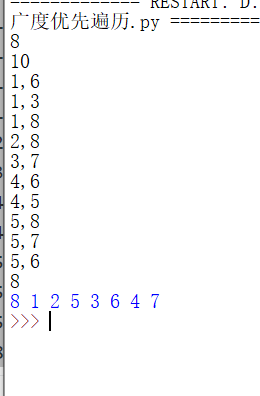深度优先遍历（DFS）
图的深度优先遍历可定义如下：首先访问出发点v，并将其标记为已访问过；然后依次从v出发搜索v的每个邻接点w。若w未曾访问过，则以w为新的出发点继续进行深度优先遍历，直至图中所有和源点v有路径相通的顶点均已被访问为止。若此时图中仍有未访问的顶点，则另选一个尚未访问的顶点为新的源点重复上述过程，直至图中所有的顶点均已被访问为止。请编写程序实现图的深度优先遍历，从节点1开始。同时由于图的深度优先遍历不唯一，我们约定对于多邻点的顶点的遍历，按顶点值由低到高进行深度优先遍历。
第一行输入图的顶点的数目；第二行输入图的边数；接下来逐行输入边。
比如：下图（还是上面那个图）输入顶点数8（对应的顶点为1~8），边数10和边集，得到的深度优先遍历的结果为：1 3 7 5 4 6 8 2class Graph(object):

def __init__(self,*args,**kwargs):
self.node_neighbors = {}
self.visited = {}

def add_nodes(self,nodelist):

for node in nodelist:
self.add_node(node)

def add_node(self,node):
if not node in self.nodes():
self.node_neighbors[node] = []

def add_edge(self,edge):
u,v = edge
if(v not in self.node_neighbors[u]) and ( u not in self.node_neighbors[v]):
self.node_neighbors[u].append(v)

if(u!=v):
self.node_neighbors[v].append(u)

def nodes(self):
return self.node_neighbors.keys()

def depth_first_search(self,root=None):
order = []
def dfs(node):
self.visited[node] = True
order.append(node)
self.node_neighbors[node].sort()
for n in self.node_neighbors[node]:
if not n in self.visited:
dfs(n)

if root:
dfs(root)

for node in self.nodes():
if not node in self.visited:
dfs(node)

#print(order)
return order

if __name__ == '__main__':
g = Graph()
node_num=int(input())#顶点的数目
#node_c=input().split(',')
#g.add_nodes(i for i in node_c)
g.add_nodes([i+1 for i in range(node_num)])
vec_num=int(input())#边的数目
for i in range(vec_num):
g.add_edge(tuple(int(n) for n in input().split(',')))

#print("nodes:", g.nodes())

order = g.depth_first_search(1)
for i in order:
print(i,end=' ')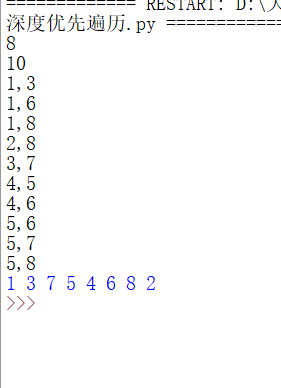最短路径树
计算最短路径树使用邻接矩阵表示较方便。它是指到某个节点路径都是最短的一棵树。下面的代码求所有节点到图的第一个节点构成的最短路径树。最短路径树使用邻接矩阵表示法较方便。第一行是图的节点数n，第二行到第n+1行，是对应的图的邻接矩阵。然后，在一行中输出从所有节点（包含第一个节点自己）到第一个节点的最短路径的长度。
n=int(input())
path_cost=[]
for i in range(n):
path_cost.append(list(map(int,input().split())))
a=+[200000000 for x in range(n-1)]
visit=[False]*n
for i in range(n):
min1=200000000
for j in range(n):
if (not visit[j]) and (a[j]<min1):
min1=a[j]
k=j
visit[k]=True
for j in range(n):
if(not visit[j]) and (a[k]+path_cost[k][j]<a[j]):
a[j]=a[k]+path_cost[k][j]
print(a)


如果两个节点之间不存在边，权重就取一个很大值，姑且输入65535。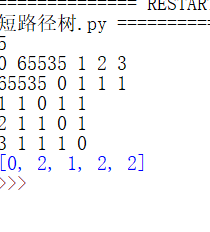最小生成树
一个有n个结点的连通图的生成树是原图的极小连通子图，且包含原图中的所有n个结点，并且有保持图连通的最少的边。
而最小生成树便是指该生成树所有边的权值之和是最小的生成树。常用的方法有prim算法和kruskal算法。
这个代码输入第一行为正整数N。（N>0）N为图的节点数，接下来输入一个N行N列的矩阵C，因为是无向图，所以这是一个沿对角线对称的矩阵，矩阵的元素Cij代表从i节点到j节点的花费值。若该条边不存在，则设置数值为65535；输出最小生成树的cost。这里给出了两种算法的函数。
class Graph(object):
def __init__(self, maps):
self.maps = maps
self.nodenum = self.get_nodenum()
self.edgenum = self.get_edgenum()

def get_nodenum(self):
return len(self.maps)

def get_edgenum(self):
count = 0
for i in range(self.nodenum):
for j in range(i):
if self.maps[i][j] > 0 and self.maps[i][j] < 9999:
count += 1
return count

def kruskal(self):
res = []
sum_cost=0
if self.nodenum <= 0 or self.edgenum < self.nodenum-1:
return res
edge_list = []
for i in range(self.nodenum):
for j in range(i,self.nodenum):
if self.maps[i][j] < 9999:
edge_list.append([i, j, self.maps[i][j]])#按[begin, end, weight]形式加入

edge_list.sort(key=lambda a:a)#已经排好序的边集合

group = [[i] for i in range(self.nodenum)]
for edge in edge_list:
for i in range(len(group)):
if edge in group[i]:
m = i
if edge in group[i]:
n = i
if m != n:
res.append(edge)
sum_cost +=edge
group[m] = group[m] + group[n]
group[n] = []
#return res
return sum_cost

def prim(self):
sum_cost=0
res = []
if self.nodenum <= 0 or self.edgenum < self.nodenum-1:
return res
res = []
seleted_node = 
candidate_node = [i for i in range(1, self.nodenum)]

while len(candidate_node) > 0:
begin, end, minweight= 0, 0, 9999
for i in seleted_node:
for j in candidate_node:
if self.maps[i][j] < minweight:
minweight = self.maps[i][j]
begin = i
end = j
res.append([begin, end, minweight])
sum_cost += minweight
seleted_node.append(end)
candidate_node.remove(end)
return sum_cost

max_value = 65535
'''
row0 = [0,7,max_value,max_value,max_value,5]
row1 = [7,0,9,max_value,3,max_value]
row2 = [max_value,9,0,6,max_value,max_value]
row3 = [max_value,max_value,6,0,8,10]
row4 = [max_value,3,max_value,8,0,4]
row5 = [5,max_value,max_value,10,4,0]
maps = [row0, row1, row2,row3, row4, row5]
maps2=[[0,6,3,2,99,99,99],
[6,0,5,99,99,2,99],
[3,5,0,3,99,1,99],
[2,99,3,0,5,99,6],
[99,99,99,5,0,6,2],
[99,2,1,99,6,0,4],
[99,99,99,6,2,4,0]]
'''
n_length=int(input())
maps=[]
for i in range(n_length):
maps.append(list(map(int,input().split())))
graph = Graph(maps)
#print('邻接矩阵为\n%s'%graph.maps)
#print('节点数据为%d，边数为%d\n'%(graph.nodenum, graph.edgenum))
#print('最小生成树kruskal算法')
#print(graph.kruskal())
#print('最小生成树prim算法')
print(graph.prim())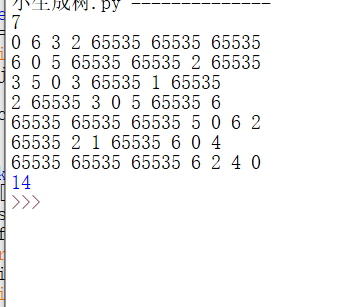展开全文算法 python
• * function:图的邻接矩阵表示，即DFS和BFS */ public class BFSVSDFS { private AdMatrixGraph graph; private int[] visited=null; private MyQueue queue=null; public BFSVSDFS(AdMatrixGraph ...算法 graph matrix function string
• DFS 算法思想 深度优先搜索思想：假设初始状态是图中所有顶点均未被访问，则从某个顶点v出发，首先访问该顶点，然后依次从它的各个未被访问的邻接点出发深度优先搜索遍历图，直至图中所有v有路径相通的顶点都被...数据结构 java
• DFS和BFS DFS（Depth-First-Search）深度优先搜索算法，是搜索算法的一种。是一种在开发爬虫早期使用较多的方法。它的目的是要达到被搜索结构的叶结点 宽度优先搜索算法（又称广度优先搜索）是最简便的图的搜索算法...
• Dijkstra单源最短路径算法Prim最小生成树算法都采用了宽度优先搜索类似的思想。 通常用队列实现，使用队列保存未被检测的节点，节点按照广度优先的次序被访问进出队列。 就像是你的眼睛被打到地上，你趴在地板...
• 2020杭电多校第六场A Very Easy Graph Problem（并查集+dfs+bfs）【最小生成树】 题目 http://acm.hdu.edu.cn/showproblem.php?pid=6832 题意 给出一个无向连通图，里面的点分为0号点1号点，第i条边的边权是2的i次...并查集
• 深度优先搜索遍历类似于的先根遍历，是的先根遍历的推广。其过程为：假设初始状态是图中所有顶点未曾被访问，则深度优先搜索可以从图中的某个顶点v出发，访问此顶点，然后依次从v的未被访问的邻接点出发深度优先...
• 文章目录代码模板BFS模板DFS模板递归玩法非递归玩法二叉树的层次遍历括号生成在每个行中找最大值岛屿数量岛屿的最大面积被围绕的区域单词接龙 代码模板 BFS模板 def BFS(graph, start, end): visited = set() ...leetcode 算法
• 树型数据结构，处理Disjoint Sets的合并查询 import java.util.Scanner; public class FindMergeSet { static int n; static int m; static int[] pre = new int; public static int find(int k){...
• 分别实现图的DFS和BFS算法(存储结构任意)。 在给定的连通网络上求解最小生成树（Prim算法和Kruscal算法任选）。 在给定的有向带权图上实现求关键路径的算法。 在带权的有向图上求解从某一原点出发到其余顶点的...数据结构 代码实现
• （1）掌握图的邻接矩阵、邻接表、十字链表等不同存储形式的表示方法。...（3）掌握构造最小生成树的两种算法，即Prim 算法Kruscal算法的思想，并能编程实现。 （4）能够灵活运用图的相关算法解决相应的实际问题。
• 其中有很多的算法都用到了这两种思想,比如:Dijkstra单源最短路径算法Prim最小生成树算法都采用了宽度优先搜索类似的思想。 BFS的思想： 从一个图的某一个顶点V0出发，首先访问V0相邻的且未被访问过的顶点V1、...
• 矩阵规则 对角线：元素 = 0 ...是否为有向图无向图 顶点数 边数 存储矩阵（python：np.narray） class Graph: def __init___(self): self.mat # 存储矩阵 self.n # 存储边数 self.type...
• DFS和BFS在图中的应用： 图连通性判定；路径的存在性；图中是否存在环；求图的最小生成树；求图的关键路径；求图的拓扑排序。 DFS：简单的说，先一直往深处走，直到不能再深了，再从另一条路开始往深处走，...
• 深度优先搜索 输入 输入的第一行包含一个正整数n，表示图中共有n个顶点。其中n不超过50。 以后的n行中每行有n个用空格隔开的整数0或...每一行输出无向图中的一棵生成树，表示按照题目描述中的深度优先遍历算法遍历相...
• 有需要关注微信公众号：算法那些事儿 ... --------------------- 咱们由BFS开始： 首先，看下算法导论一书关于 此BFS 广度优先搜索算法的概述。...算法导论第二版，中译本，第324页。...在Prime最小生成树算法，D...
• 图论中的优先级搜索——DFS,BFS,Prim,Dijkstra  在图算法中经常要执行遍历每个顶点每条边的操作，即图搜索。许多图算法都以图搜索为基础，如2-着色问题、...基于搜索的算法还包括计算最小生成树的Prim算法
• 文章目录图 Graph一、图的连通性1.... 深度搜索广度搜索问题6.无向图下的最短路径问题7.巧妙的构建DAG 图 Graph 一、图的连通性 1. 宽度优先搜索 BFS 基本思路：先选择一个点作为起始点，可以将这个起始点作为算法 有向图
• 最小生成树算法、Dijkstra最短路径算法都采用了和BFS相似的思想。广度优先搜索和二叉树的层序遍历较为相似，需要借助于队列实现；深度优先搜索和二叉树的前序遍历较为相似，需要借助于栈实现；无论是BFS还是DFS，都...数据结构 算法
• 所示的有向图G的邻接矩阵，并分别输出顶点表邻接矩阵。 在图G的邻接矩阵存储表示基础上，实现深度优先遍历算法，输出从顶点V1开始的深度优先遍历序列。 实现广度优先遍历算法，输出从顶点V1开始的广度优先遍历序列...数据结构
• 通过遍历构造生成树的过程可以按深度优先方式或宽度优先方式进行，在遍历中记录访问的所有顶点经过的边，就得到原图的深度优先生成树（简称DFS生成树），或者宽度优先生成树(BFS生成树）。 在得到的生成树中，每...
• 最小生成树 Prim 基本思路：将点的集合分为C V-C ，分别为访问过的。 Krusal 将每个顶点维护成单顶点连通分量C(v1),…C(vn) C(v_1),…C(v_n) 1. 先将边进行排序 2. 每次加入权值最小的边，如果两...prim 搜索
• 前言这几天复习图论算法，觉得BFS和DFS挺重要的，而且应用比较多，故记录一下。广度优先搜索有一个有向图如图a 图a 广度优先搜索的策略是：从起始点开始遍历其邻接的节点，由此向外不断扩散。1.假设我们以顶点0为......